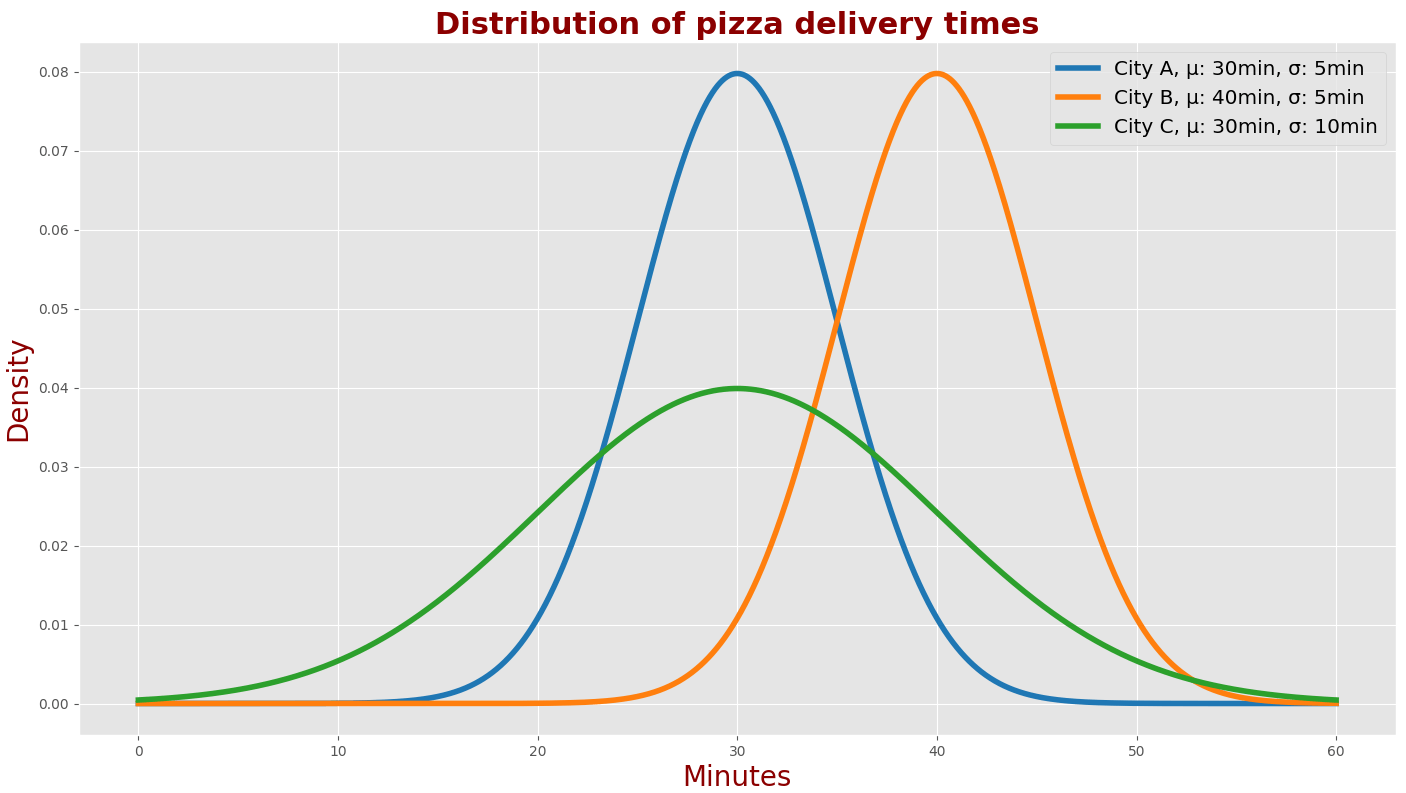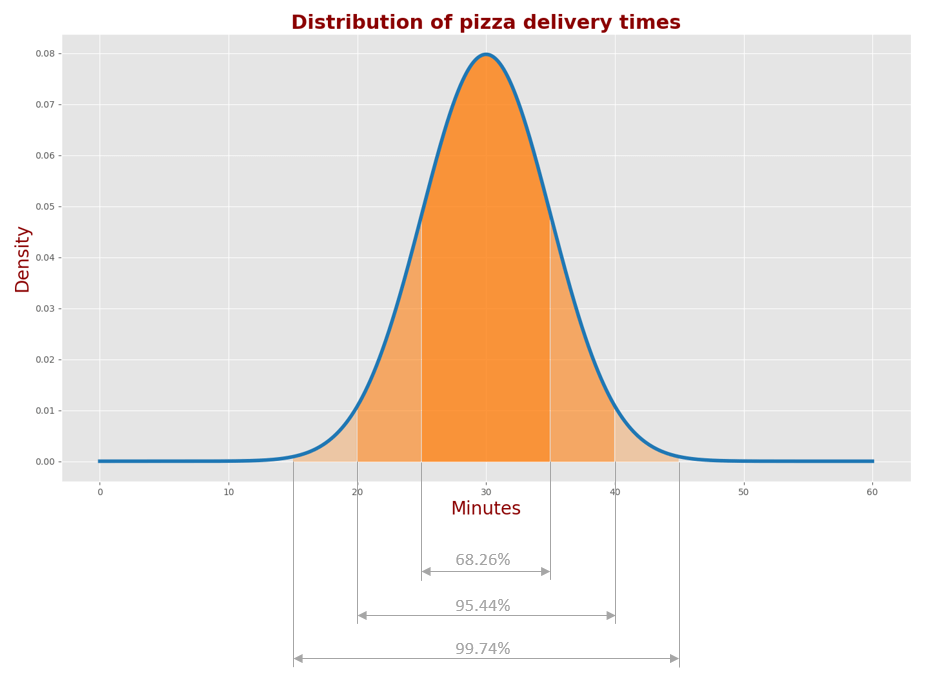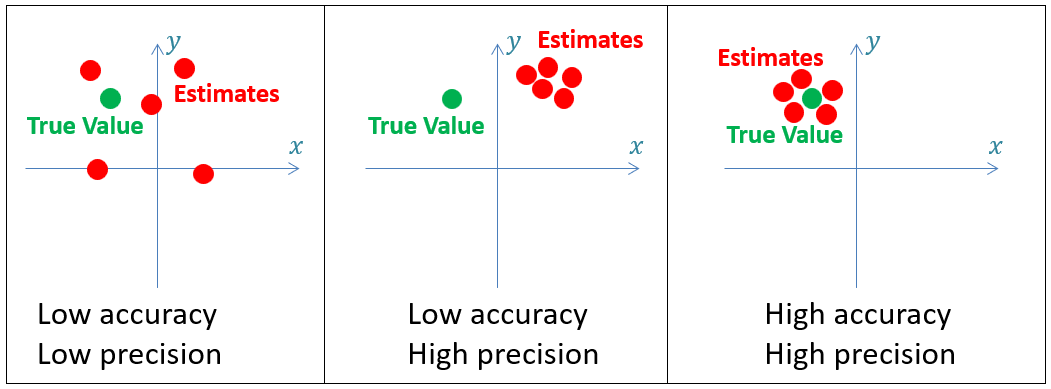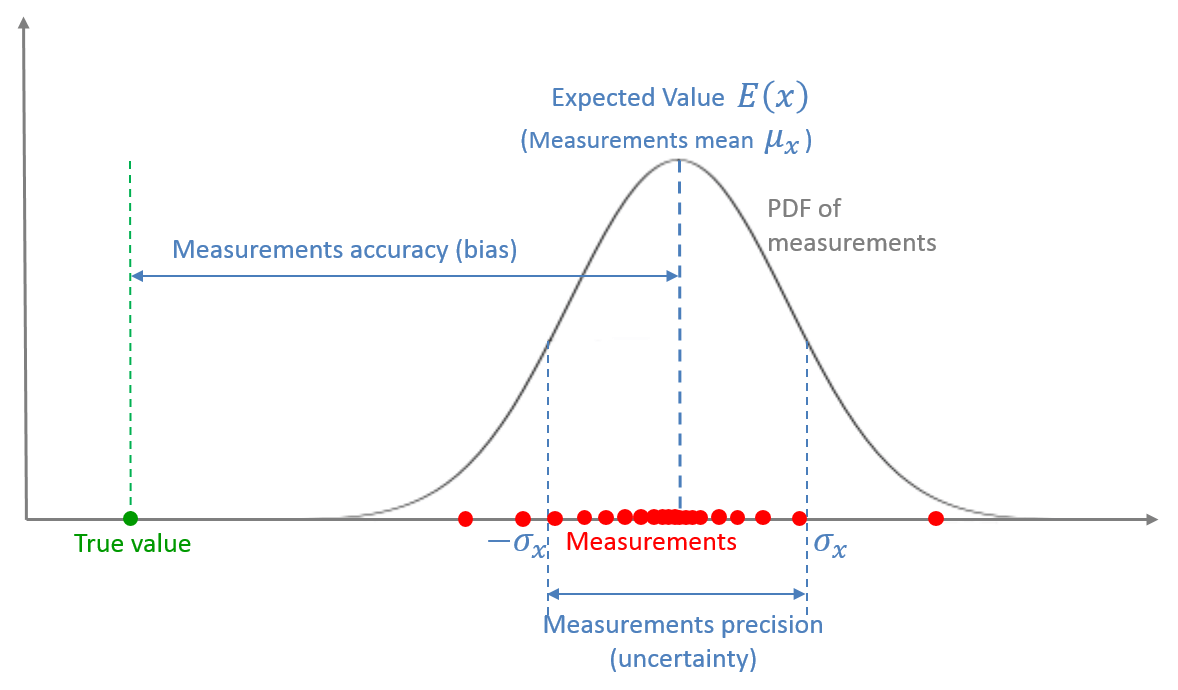﻿ Essential background I

# Essential background I

Before we start, I would like to explain several fundamental terms such as variance, standard deviation, normal distribution, estimate, accuracy, precision, mean, expected value, and random variable.

I expect that many readers of this tutorial are familiar with introductory statistics. However, at the beginning of this tutorial, I promised to supply the necessary background that is required to understand how the Kalman Filter works. If you are familiar with this topic, feel free to skip this chapter and jump to the next section.

## Mean and Expected Value

Mean and Expected Value are closely related terms. However, there is a difference.

For example, given five coins – two 5-cent coins and three 10-cent coins, we can easily calculate the mean value by averaging the values of the coins.$V_{mean}= \frac{1}{N} \sum _{n=1}^{N}V_{n}= \frac{1}{5} \left( 5+5+10+10+10 \right) = 8cent$

The above outcome cannot be defined as the expected value because the system states (the coin values) are not hidden, and we've used the entire population (all 5 coins) for the mean value calculation.

Now assume five different weight measurements of the same person: 79.8kg, 80kg, 80.1kg, 79.8kg, and 80.2kg. The person is a system, and the person's weight is a system state.The measurements are different due to the random measurement error of the scales. We do not know the true value of the weight since it is a Hidden State. However, we can estimate the weight by averaging the scales' measurements.

$W = \frac{1}{N} \sum _{n=1}^{N}W_{n}= \frac{1}{5} \left( 79.8+80+80.1+79.8+80.2 \right) = 79.98kg$

The outcome of the estimate is the expected value of the weight.

The expected value is the value you would expect your hidden variable to have over a long time or many trials.

The mean is usually denoted by the Greek letter μ.

The letter E usually denotes the expected value.

## Variance and Standard deviation

The Variance is a measure of the spreading of the data set from its mean.

The Standard Deviation is the square root of the variance.

The standard deviation is denoted by the Greek letter $$\sigma$$ (sigma). Accordingly, the variance is denoted by $$\sigma^{2}$$.

Suppose we want to compare the heights of two high school basketball teams. The following table provides the players' heights and the mean height of each team.

Player 1 Player 2 Player 3 Player 4 Player 5 Mean
Team A 1.89m 2.1m 1.75m 1.98m 1.85m 1.914m
Team B 1.94m 1.9m 1.97m 1.89m 1.87m 1.914m

As we can see, the mean height of both teams is the same. Let us examine the height variance.

Since the variance measures the spreading of the data set, we would like to know the data set deviation from its mean. We can calculate the distance from the mean for each variable by subtracting the mean from each variable.

The height is denoted by $$x$$, and the heights mean by the Greek letter $$\mu$$. The distance from the mean for each variable would be:

$x_{n} - \mu = x_{n}-1.914m$

The following table presents the distance from the mean for each variable.

Player 1 Player 2 Player 3 Player 4 Player 5
Team A -0.024m 0.186m -0.164m 0.066m -0.064m
Team B 0.026m -0.014m 0.056m -0.024m -0.044m

Some of the values are negative. To get rid of the negative values, let us square the distance from the mean:

$\left( x_{n}- \mu \right) ^{2} = \left( x_{n}- 1.914m \right) ^{2}$

The following table presents the squared distance from the mean for each variable.

Player 1 Player 2 Player 3 Player 4 Player 5
Team A 0.000576m2 0.034596m2 0.026896m2 0.004356m2 0.004096m2
Team B 0.000676m2 0.000196m2 0.003136m2 0.000576m2 0.001936m2

To calculate the variance of the data set, we need to find the average value of all squared distances from the mean:

$\sigma ^{2}= \frac{1}{N} \sum _{n=1}^{N} \left( x_{n}- \mu \right) ^{2}$

For team A, the variance would be:

$\sigma _{A}^{2} = \frac{1}{N} \sum _{n=1}^{N} \left( x_{n}- \mu \right) ^{2}= \frac{1}{5} \left( 0.000576+ 0.034596+ 0.026896+ 0.004356+ 0.004096 \right) = 0.014m^{2}$

For team B, the variance would be:

$\sigma _{B}^{2} = \frac{1}{N} \sum _{n=1}^{N} \left( x_{n}- \mu \right) ^{2}= \frac{1}{5} \left( 0.000676+ 0.000196+ 0.003136+ 0.000576+ 0.001936 \right) = 0.0013m^{2}$

We can see that although the mean of both teams is the same, the measure of the height spreading of Team A is higher than the measure of the height spreading of Team B. Therefore, the Team A players are more diverse than the Team B players. There are players for different positions like ball handler, center, and guards, while the Team B players are not versatile.

The units of the variance are meters squared; it is more convenient to look at the standard deviation, which is a square root of the variance.

$\sigma =\sqrt[]{\frac{1}{N} \sum _{n=1}^{N} \left( x_{n}- \mu \right) ^{2}}$

The standard deviation of Team A players' heights would be 0.12m.

The standard deviation of Team B players' heights would be 0.036m.

Now, assume that we would like to calculate the mean and variance of all basketball players in all high schools. That would be an arduous task - we would need to collect data on every player from every high school.

On the other hand, we can estimate the players' mean and variance by picking a big data set and making the calculations on this data set.

The data set of 100 randomly selected players should be sufficient for an accurate estimation.

However, when we estimate the variance, the equation for the variance calculation is slightly different. Instead of normalizing by the factor $$N$$, we shall normalize by the factor $$N-1$$:

$\sigma_{sampled}^{2} = \frac{1}{N-1} \sum _{n=1}^{N} \left( x_{n}- \mu \right) ^{2}$

The factor of $$N-1$$ is called Bessel's correction.

You can see the mathematical proof of the above equation on visiondummy or Wikipedia.

## Normal Distribution

It turns out that many natural phenomena follow the Normal Distribution. The normal distribution, also known as the Gaussian (named after the mathematician Carl Friedrich Gauss), is described by the following equation:

$f \left( x; \mu , \sigma ^{2} \right) = \frac{1}{\sqrt[]{2 \pi \sigma ^{2}}}e^{\frac{- \left( x- \mu \right) ^{2}}{2 \sigma ^{2}}}$

The Gaussian curve is also called the Probability Density Function (PDF) for the normal distribution.

The following chart describes PDFs of the pizza delivery time in three cities: city 'A,' city 'B,' and city 'C.'• In city 'A,' the mean delivery time is 30 minutes, and the standard deviation is 5 minutes.
• In city 'B,' the mean delivery time is 40 minutes, and the standard deviation is 5 minutes.
• In city 'C,' the mean delivery time is 30 minutes, and the standard deviation is 10 minutes.

We can see that the Gaussian shapes of the city 'A' and city 'B' pizza delivery times are identical; however, their centers are different. That means that in city 'A,' you wait for pizza for 10 minutes less on average, while the measure of spread in pizza delivery time is the same.

We can also see that the centers of Gaussians in the city 'A' and city 'C' are the same; however, their shapes are different. Therefore the average pizza delivery time in both cities is the same, but the measure of spread is different.

The following chart describes the proportions of the normal distribution.• 68.26% of the pizza delivery times in City A lie within $$\mu \pm \sigma$$ range (25-35 minutes)
• 95.44% of the pizza delivery times in City A lie within $$\mu \pm 2\sigma$$ range (20-40 minutes)
• 99.74% of the pizza delivery times in City A lie within $$\mu \pm 3\sigma$$ range (15-45 minutes)

Usually, measurement errors are distributed normally. The Kalman Filter design assumes a normal distribution of the measurement errors.

## Random Variables

A random variable describes the hidden state of the system. A random variable is a set of possible values from a random experiment.

The random variable can be continuous or discrete:

• A continuous random variable can take any value within a specific range, such as battery charge time or marathon race time.
• A discrete random variable is countable, such as the number of website visitors or the number of students in the class.

The random variable is described by the probability density function. The probability density function is characterized by moments.

The moments of the random value are expected values of powers of the random variable. We are interested in two types of moments:

• The $$k^{th}$$ raw moment is the expected value of the $$k^{th}$$ power of the random variable: $$E\left( X^{k} \right)$$.
• The $$k^{th}$$ central moment is the expected value of the $$k^{th}$$ power of the random variable distribution about its mean: $$E\left( \left( X - \mu_{X} \right)^{k} \right)$$.

In this tutorial, the random variables are characterized by the following:

• The first raw moment $$E\left( X \right)$$ – the mean of the sequence of measurements.
• The second central moment $$E\left( \left( X - \mu_{X} \right)^{2} \right)$$ – the variance of the sequence of measurements.

## Estimate, Accuracy and Precision

An Estimate is about evaluating the hidden state of the system. The true position of the aircraft is hidden from the observer. We can estimate the aircraft position using sensors, such as radar. The estimate can be significantly improved by using multiple sensors and applying advanced estimation and tracking algorithms (such as the Kalman Filter). Every measured or computed parameter is an estimate.

Accuracy indicates how close the measurement is to the true value.

Precision describes the variability in a series of measurements of the same parameter. Accuracy and precision form the basis of the estimate.

The following figure illustrates accuracy and precision.High-precision systems have low variance in their measurements (i.e., low uncertainty), while low-precision systems have high variance in their measurements (i.e., high uncertainty). The random measurement error produces the variance.

Low-accuracy systems are called biased systems since their measurements have a built-in systematic error (bias).

The influence of the variance can be significantly reduced by averaging or smoothing measurements. For example, if we measure temperature using a thermometer with a random measurement error, we can make multiple measurements and average them. Since the error is random, some measurements would be above the true value and others below the true value. The estimate would be close to the true value. The more measurements we make, the closer the estimate will be.

On the other hand, a biased thermometer produces a constant systematic error in the estimate.

All examples in this tutorial assume unbiased systems.

## Summary

The following figure represents a statistical view of measurement.A measurement is a random variable, described by the Probability Density Function (PDF).

The mean of the measurements is the Expected Value of the random variable.

The offset between the mean of the measurements and the true value is the accuracy of the measurements, also known as bias or systematic measurement error.

The dispersion of the distribution is the measurement precision, also known as the measurement noise, random measurement error, or measurement uncertainty.